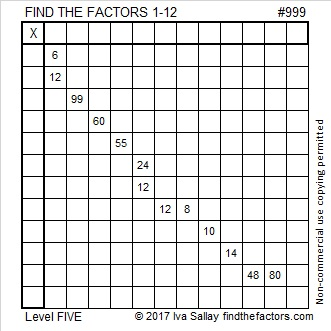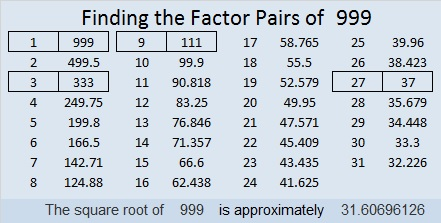# 999 and Level 5

What are the common factors of 6 and 12? There are many: 1, 2, 3, and 6 are all common factors and possibilities for this puzzle. Only one of those numbers will work with the other clues to make this puzzle a multiplication table. That why 6 and 12 is NOT a good place to start when solving this puzzle. Look at all the other rows and columns with two or more clues. ONE of them will be the perfect place to start. Good luck!Print the puzzles or type the solution in this excel file: 12 factors 993-1001

999 is the smallest number whose digits add up to 27.

999 is 666 upside-down.

Did you know that 999² = 998001? That’s cool because 998 + 001 = 999. Numbers whose squares can be separated and then added together to get the original number are so unusual, they’ve been given a name, Kaprekar numbers. There are only seven Kaprekar numbers less than 999. OEIS.org was my source for this fun 999 fact.

999 is the hypotenuse of a Pythagorean triple:
324-945-999 which is 27 times (12-35-37)

999 is a repdigit in base 10 and it looks interesting in some other bases, too:
It’s 4343 in BASE 6 because 4(6³) + 3(6²) + 4(6¹) + 3(6⁰) = 999,
It’s palindrome 515 in BASE 14 because 5(14²) + 1(14) + 5(1) = 999,
and it’s repdigit RR in BASE 36 (R is 27 base 10) because 27(36) + 27(1) = 27(37) = 999

• 999 is a composite number.
• Prime factorization: 999 = 3 × 3 × 3 × 37, which can be written 999 = 3³ × 37
• The exponents in the prime factorization are 3 and 1. Adding one to each and multiplying we get (3 + 1)(1 + 1) = 4 × 2 = 8. Therefore 999 has exactly 8 factors.
• Factors of 999: 1, 3, 9, 27, 37, 111, 333, 999
• Factor pairs: 999 = 1 × 999, 3 × 333, 9 × 111, or 27 × 37
• Taking the factor pair with the largest square number factor, we get √999 = (√9)(√111) = 3√111 ≈ 31.60696